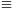Mathematical and Physical Journal
for High Schools
Issued by the MATFUND Foundation
 Already signed up? New to KöMaL?

#Problem A. 545. (November 2011)

A. 545. Prove that whenever a>b>1 are integers such that a+b divides ab+1 and a-b divides ab-1 then.

(Kolmogorov Cup, 2009)

(5 pont)

Deadline expired on December 12, 2011.

Solution. Since b2-1=b(a+b)-(ab+1)0 (mod  (a+b)) and b2-1=-b(a-b)+(ab-1)0 (mod  (a-b)), both a+b and a-b divide (b2-1). Hence, b2-1 is divisible by the least common multiple of a+b and a-b. By the conditions b2-1 and a-b are positive, so this implies

 [a+b,a-b]b2-1. (1)

Next we show that a and b are coprime; moreover, the greatest common divisor of a+b and a-b is at most 2. Let d=(a,b). Then d|a|ab and d|a+b|ab+1, so d|(ab+1)-ab=1 and thus d=1. Now let e=(a+b,a-b). Then by e|(a+b)+(a-b)=2a and e|(a+b)-(a-b)=2b we have e|(2a,2b)=2(a,b)=2. Therefore, e2.

From the identitywe getCombining this with (1), we geta23b2-2<3b2### Statistics:

 9 students sent a solution. 5 points: Ágoston Tamás, Gyarmati Máté, Janzer Olivér, Kovács 444 Áron, Mester Márton, Omer Cerrahoglu, Strenner Péter, Szabó 928 Attila. 0 point: 1 student.

Problems in Mathematics of KöMaL, November 2011